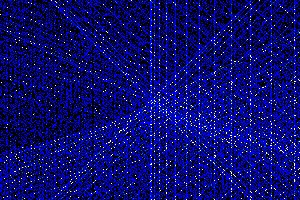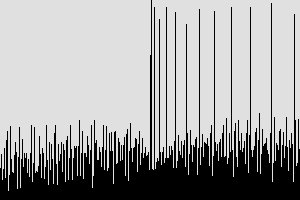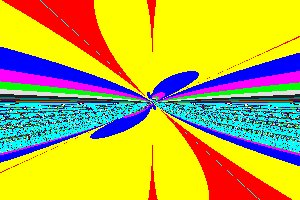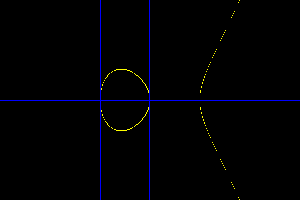## elliptic curves

Return

This file was generated from ec.txt

#### ILLUSTRATED NOTES ON ELLIPTIC CURVESThe first image is called elliptic curve spiral. Each pixel at position (x,y) is colored according to then number of solutions of the equation T2 = S3+yS+x. The image is obtained by generating a sequence of planes paramaterised by values of S. A dot is placed at (x,y) in each S-plane whenever S3+yS+x is a perfect square . The resulting image is obtained by adding the pixels of each S-plane, and colorizing. The image summarizes basic information for about quarter of a million elliptic curves.

The first time I calculated this image, back in 2004,I was unable to view it. The calculation took several hours, mostly because I was using a slow algorithm. After a long wait I typed in the command "display ec0.xpm &" and the computer froze. There was no response from either the keyboard and mouse and even the kill combination CTRL-ALT-DEL failed. I had to switch off the power and reboot. The small memory size of the computer along with the 2004 versions of ImageMacick and Linux were not up to displaying the image. As a result I called the first version of the image "paralyze.gif"

In 2009 energy saving features in Linux make it hard to run programs for several hours, because the machine hibernates after quite a short period of user inactivity. Faster machines and better programming techniques make this point counting calculation reasonably fast even for a larger image size.Each 3x3 tile in the image must contain one blank point. This can easily be proved by looking at the set of all curves t2=s3+sx+y (mod 3).

The vertical bars to the right correspond to values x = n2 or the square numbers. They express the fact that t2=s3+as+n2 always has a point (n,0), whatever the value of a. The slanting lines satisfy the equations sy+x = t2-s3 and sy-x = t2-s3, or y= +- x/k + (t2-k3)/k. When k>1 the pixels on the sloping line become rather spread out, compared to points that would be placed using a computer line drawing algorithm. Areas of black correspond to elliptic curves where there are no integer solutions for the checked range. A frequency count for vertical lines shows sharp spikes at the points x=n2.The equations X3+aX+b = 0 may be solved by the Newton Raphson process. This gives a sequence

X[n+1] <- (2X[n]^3-b)/(3X[n]^2+a)

The fractal like image colours each pixel according to the rate of convergence, for each equation with paramaters (a,b)=(y,x).

There are many questions arising from collections of curves like this. In the 1920's Mordell wrote a whole book about an equation such as y2=x3+6 which apparently has no integer solutions at all. It was known since the early 1800s that points on these curves formed a group under a law of composition which is simple to describe, but hard to calculate. In the theorem named after him, Mordell proved that the rational points on such a curve formed an Abelian group with a torsion group T and r copies of the integers (Z). A subgroup T of an abelian group T is called a torsion group if and only if for each t in T there exists a number n = order(t) such that n.t = 0. n.t is the element obtained by adding t to itself n times. The number 'r' is called the rank of an elliptic curve. The calculation of the group for a curve is tricky. The same applies when the curve is in a large finite field. In the latter case group addition may be used as a basis for cryptographic systems which avoid the RSA algorithm of Rivest, Shamir, Adelman. Elliptic curve cryptography was all the rage for early mobile phones systems in the 1990s. Another thing that has stimulated research in this area was Wiles search for a proof of Fermat's Last Theorem (FLT). Such a proof was found, but the mathemtics is not very accessible to beginners.

#### CHORD TANGENT PROCESSThe images demonstrate the chord tangent process. A particularly simple example is shown, because it is easy to plot. The vertical lines are tangents to the curve, and the horizontal lines joint the three roots of y2=x3-x with y=0. Together these three points represent generators of the group Z2xZ2. This is a torsion group with four elements.

It is possible to define a group operation on any curve E:y2=x3+ax+b. Given any pair of points (x1,y1) and (x2,y2) the equation of the line joining the points is y=mx+c with m=(y2-y1)/(x2-x1). Substituting this in E gives:-

(mx+c)2 = x3 + ax + b
This can be rearranged into a cubic equation with 3 roots.
x3 - m2x2 + Ax +B

Two of the roots are x0, and x1 and the third root x2 can be found from the fact that the coefficient of x2 is the sum of the roots.

x0+x1+x2 = m2
x2 = m2 - (x1+x2)
A corresponding y value can be found by putting
y2 = -(m.x2 + c)
where c = y0 - m.x1

When x0=x1, then m is computed as the slope of the tangent at x0 or dy/dx[x=x0]. Here dy/dx is evaluated from the formula

2 dy/dx = 3x2+a or dy/dx = (3x2+a)/2y

When y0=y1 or x0=x1 and y0=0, then the new value (x2,y2) is written as (1,0). The chord tangent process defines an operation on a pair of points P=(x0,y0) and Q=(x1,y1) written P+Q. 2.P is P+P and 3.P is 2.P+P or P+2.P and so on. It can be shown, with some difficulty, that the set of points P=(x,y) along with (1,0) along with the operation '+' satisfy the axioms of an Abelian Group. The point 0=(1,0) is the zero in the group and -P = (x,-y) where P = (x,y). The difficulty arises in proving associative law (P+Q)+R = P+(Q+R) for three pairs of points, P,Q, and R. Silverman suggests using a computer algebra package to show that a repeated chord tangent process generates a symetric formula in six variables x1,x2,x3,y1,y2,y3. There is some merit in this approach.

#### IMPORTANT CONCEPTS

Chinese Remainder Theorem, Diaphontine Equations, Abelian Groups, Convergence and Limits, Rings and Fields.